# 1999 seminars

## 04/02/2020, Tuesday, 15:00–16:00

Luís Diogo, Universidade Federal Fluminense, Brasil
Lifting Lagrangians from symplectic divisors

We prove that there are infinitely many non-symplectomorphic monotone Lagrangian tori in complex projective spaces, quadrics and cubics of complex dimension at least $3$. This is a consequence of the following: if $Y$ is a codimension $2$ symplectic submanifold of a closed symplectic manifold $X$, then we can explicitly relate the superpotential of a monotone Lagrangian $L$ in $Y$ with the superpotential of a monotone Lagrangian lift of $L$ in $X$. This sometimes involves relative Gromov-Witten invariants of the pair $(X,Y)$. We will define the superpotential, which is a count of pseudoholomorphic disks with boundary on a Lagrangian, and which plays an important role in Floer theory and mirror symmetry. This is joint work with D. Tonkonog, R. Vianna and W. Wu.

## 12/02/2020, Wednesday, 15:00–16:00

Lucas Ambrozio, University of Warwick
Sharp inequalities relating volume and the min-max widths of Riemannian three-spheres.

Min-max theories for the area functional have undergone ground-break developments in recent years. One aspect of these theories is that they define many notions of "width" that can be understood as geometric invariants of compact Riemannian manifolds. As such, it is an interesting question to understand what sort of geometric information they encode. In this talk, we will focus on the Simon-Smith width of Riemannian three-dimensional spheres, discussing how large it can be among metrics normalised to have the same volume, and necessary conditions for maxima under further restrictions. This is a joint work with Rafael Montezuma (UM-Amherst).

## 19/02/2020, Wednesday, 15:00–16:00

Helge Ruddat, Gutenberg University
Finite order period integrals in normal crossing K3 degenerations

I am presenting a new technique to compute period integrals of degenerating Calabi-Yau manifolds. In the K3 case, the formula can be used to study the Picard group of nearby fibres. In general, the technique leads to canonical parametrizations of degenerating families at the boundary of the moduli space. The result enters ongoing work by Gross-Hacking-Keel-Siebert on a modular compactification of the family of g-polarized K3 surfaces. This is joint with Bernd Siebert.

## 21/02/2020, Friday, 15:30–16:30

Special Lagrangians, Lagrangian mean curvature flow and the Gibbons-Hawking ansatz

(all joint work with Jason Lotay) A standing conjecture of Richard Thomas, motivated by mirror symmetry, gives a stability condition supposed to control the existence of a special Lagrangian submanifold in a given Hamiltonian isotopy class of Lagrangians. Later, Thomas and Yau conjectured a similar stability condition controls the long-time existence of the Lagrangian mean-curvature flow. In this talk I will explain how Jason Lotay and myself have recently proved versions of these conjectures on all circle symmetric hyperKahler 4-manifolds.

## 05/05/2020, Tuesday, 17:00–18:00 Europe/Lisbon — Online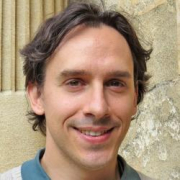Alexander Ritter, University of Oxford
Invariance of symplectic cohomology under deformations

This is joint work with Gabriele Benedetti (Heidelberg).

I will describe how Floer cohomology changes as one deforms the symplectic form. I will then explain how these results are useful in applications in symplectic topology, e.g. finding generators for the Fukaya category of toric varieties or finding lower bounds on the number of magnetic geodesics.

Ritter Slides - Lisbon 5 May 2020.pdf

## 12/05/2020, Tuesday, 17:00–18:00 Europe/Lisbon — OnlineRui Loja Fernandes, University of Illinois at Urbana-Champaign
Non-commutative integrable systems and their singularities

The theory of singularities of non-commutative integrable systems (a.k.a isotropic fibrations), in contrast with the well-known theory for the commutative case (a.k.a. Lagrangian fibrations), is nonexistent. In this talk I will describe a few first steps toward such a theory.

Rui_slides.pdf

## 19/05/2020, Tuesday, 16:30–17:30 Europe/Lisbon — Online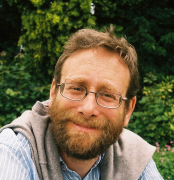Complete hyperKaehler 4-manifolds with cubic volume growth (and suitable decay of the curvature), also known as ALF gravitational instantons, are known to come in two families, according to the fundamental group at infinity. This group must be a finite subgroup of $SU(2)$ and the only possibilities compatible with cubic volume growth are the cyclic groups ($A_k$) and binary dihedral groups ($D_k$).

This talk will be about the construction of $D_k$ ALF gravitational instantons by a gluing construction in which the ingredients are the moduli space of centred charge-2 monopoles ($D_0$) and a particularly symmetric, but singular, $A_k$ ALF gravitational instanton. This construction was suggested in a paper of Sen (1997). It is also closely related to a construction due to Foscolo, in which hyperKaehler metrics are constructed on the $K3$ manifold that are “nearly” collapsed to a 3-dimensional space.

This is joint work with Bernd Schroers.

## 26/05/2020, Tuesday, 17:00–18:00 Europe/Lisbon — Online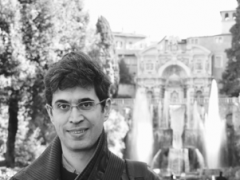Xavier Roulleau, Université d’Aix-Marseille
On a special configuration of $12$ conics and a related $K3$ surface

A generalized Kummer surface $X$ obtained as the quotient of an abelian surface by a symplectic automorphism of order 3 contains a $9{\mathbf A}_{2}$-configuration of $(-2)$-curves (ie smooth rational curves). Such a configuration plays the role of the $16$ disjoint $(-2)$-curves for the usual Kummer surfaces.

In this talk we will explain how construct $9$ other such $9{\mathbf A}_{2}$-configurations on the generalized Kummer surface associated to the double cover of the plane branched over the sextic dual curve of a cubic curve.

The new $9{\mathbf A}_{2}$-configurations are obtained by taking the pullback of a certain configuration of $12$ conics which are in special position with respect to the branch curve, plus some singular quartic curves. We will then explain how construct some automorphisms of the K3 surface sending one configuration to another.

(Joint work with David Kohel and Alessandra Sarti).

## 02/06/2020, Tuesday, 17:00–18:00 Europe/Lisbon — OnlineSteve Zelditch, Northwestern University
Probabilistic aspects of toric Kahler geometry

Let $(M, \omega, L)$ be a polarized toric Kahler manifold with polytope $P$. Associated to this data is a family $\mu_k^x$ of probability measures on $P$ parametrized by $x \in P.$ They generalize the multi-nomial measures on the simplex, where $M = \mathbb{CP}^n$ and $\omega$ is the Fubini-Study measure. As is well-known, these measures satisfy a law of large numbers, a central limit theorem, a large deviations principle and entropy asymptotics. The measure of maximal entropy in this family corresponds to the center of mass $x$ of $P$. All of these results generalize to any toric Kahler manifold, except the center of mass result, which holds for Fano toric Kahler-Einstein manifolds.

Joint work with Peng Zhou and Pierre Flurin.

### Video

Zelditch_slides.pdf

## 09/06/2020, Tuesday, 17:00–18:00 Europe/Lisbon — OnlineKai Cieliebak, Augsburg University
Partial orders on contactomorphism groups and their Lie algebras

Eliashberg, Kim and Polterovich constructed nontrivial partial orders on contactomorphism groups of certain contact manifolds. After recalling their results, the subject of this talk will be the remnants of these partial orders on the orbits of the coadjoint action on their Lie algebras.

### Video

Cieliebak_slides_with_notes_from_talk.pdf

## 16/06/2020, Tuesday, 17:00–18:00 Europe/Lisbon — OnlineAlessia Mandini, IST and Universidade Federal Fluminense
Quasi-parabolic Higgs bundles and null hyperpolygon spaces

Hyperpolygons spaces are a family of hyperkähler manifolds, that can be obtained from coadjoint orbits by hyperkähler reduction. Jointly with L. Godinho, we showed that these space are isomorphic to certain families of parabolic Higgs bundles, when a suitable condition between the parabolic weights and the spectra of the coadjoint orbits is satisfied.

In analogy to this construction, we introduce two moduli spaces: the moduli spaces of quasi-parabolic $\operatorname{SL}(2,\mathbb{C})$-Higgs bundles over $\mathbb{CP}^1$ on one hand and the null hyperpolygon spaces on the other, and establish an isomorphism between them.

Finally we describe the fixed loci of natural involutions defined on these spaces and relate them to the moduli space of null hyperpolygons in the Minkowski $3$-space.

This is based in joint works with Leonor Godinho.

### Video

Mandini_slides.pdf

## 23/06/2020, Tuesday, 17:00–18:00 Europe/Lisbon — OnlineGauge theory for string algebroids

In this talk I will overview recent joint work with Roberto Rubio and Carl Tipler in arXiv:2004.11399. We introduce a moment map picture for string algebroids, a special class of holomorphic Courant algebroids introduced in arXiv:1807.10329. An interesting feature of our construction is that the Hamiltonian gauge action is described by means of Morita equivalences, as suggested by higher gauge theory. The zero locus of the moment map is given by the solutions of the Calabi system, a coupled system of equations which provides a unifying framework for the classical Calabi problem and the Hull-Strominger system. Our main results are concerned with the geometry of the moduli space of solutions. Assuming a technical condition, we prove that the moduli space carries a pseudo-Kähler metric with Kähler potential given by the dilaton functional, a topological formula for the metric, and an infinitesimal Donaldson-Uhlenbeck-Yau type theorem. Finally, we relate our topological formula to a physical prediction for the gravitino mass in order to obtain a new conjectural obstruction for the Hull-Strominger system.

### Video

Garcia-Fernandez.pdf

## 30/06/2020, Tuesday, 17:00–18:00 Europe/Lisbon — OnlineTara Holm, Cornell University
Symplectic embeddings and infinite staircases

McDuff and Schlenk determined when a four-dimensional symplectic ellipsoid can be symplectically embedded into a four-dimensional ball. They found that if the ellipsoid is close to round, the answer is given by an infinite staircase determined by Fibonacci numbers, while if the ellipsoid is sufficiently stretched, all obstructions vanish except for the volume obstruction. Infinite staircases have also been found when embedding ellipsoids into polydisks (Frenkel-Muller, Usher) and into the ellipsoid $E(2,3)$ (Cristofaro-Gardiner-Kleinman). We will describe a general approach to the question of when embedding ellipsoids into a toric target has an infinite staircase, where we provide the first obstruction to the existence of a staircase. We use this obstruction to explore infinite staircases for toric symplectic manifolds, identifying three new infinite staircases, and culminating in the conjecture that these are the only toric examples. We will describe further work-in-progress on ellipsoid embedding functions with more general targets. I will not assume any prior acquaintance with infinite staircases and will motivate the talk with plentiful examples and pictures. This talk is based on a number of collaborations with Dan Cristofaro-Gardiner, Alessia Mandini, and Ana Rita Pires; Maria Bertozzi, Emily Maw, Dusa McDuff, Grace Mwakyoma, Ana Rita Pires, Morgan Weiler; and Nicki Magill.

### Video

Slides of the talk

## 07/07/2020, Tuesday, 17:00–18:00 Europe/Lisbon — OnlineThe moduli of $(C,f)$ where $C$ is a curve and $f$ is a rational function leads to the well-developed theory of Hurwitz spaces. The study of the moduli of $(C,\omega)$ where $C$ is a curve and $\omega$ is a meromorphic differential is a younger subject. I will discuss recent developments in the study of the moduli spaces of holomorphic/meromorphic differentials on curves. Many of the basic questions about cycle classes and integrals have now been solved (through the work of many people) — but there are also several interesting open directions.

### Video

Pandharipande_slides.pdf

## 14/07/2020, Tuesday, 17:00–18:00 Europe/Lisbon — OnlineTian-Jun Li, University of Minnesota
Symplectic rational $G$-surfaces and the plane Cremona group

We give characterizations of a finite group $G$ acting symplectically on a rational surface ($\mathbb{CP}^2$ blown up at two or more points). In particular, we obtain a symplectic version of the dichotomy of $G$-conic bundles versus $G$-del Pezzo surfaces for the corresponding $G$-rational surfaces, analogous to the one in algebraic geometry. The connection with the symplectic mapping class group will be mentioned.

This is a joint work with Weimin Chen and Weiwei Wu (and partly with Jun Li).

Li_TJ_slides.pdf

## 21/07/2020, Tuesday, 17:00–18:00 Europe/Lisbon — OnlineColin Guillarmou, Laboratoire de Mathématiques d'Orsay, Université Paris-Sud
On the marked length spectrum and geodesic stretch in negative curvature

I will review a couple of recent of results proved with T. Lefeuvre and G. Knieper on the local rigidity of the marked length spectrum of negatively curved metrics.

### Video

Guillarmou_slides.pdf

## 28/07/2020, Tuesday, 17:00–18:00 Europe/Lisbon — OnlineMark Gross, Department of Pure Mathematics and Mathematical Statistics, University of Cambridge
Intrinsic Mirror Symmetry

I will talk about joint work with Bernd Siebert, proposing a general mirror construction for log Calabi-Yau pairs, i.e., a pair $(X,D)$ with $D$ a “maximally degenerate” boundary divisor and $K_X+D=0$, and for maximally unipotent degenerations of Calabi–Yau manifolds. We accomplish this by constructing the coordinate ring or homogeneous coordinate ring respectively in the two cases, using certain kinds of Gromov-Witten invariants we call “punctured invariants”, developed jointly with Abramovich and Chen.

Gross_slides.pdf

## 08/09/2020, Tuesday, 17:00–18:00 Europe/Lisbon — OnlineNick Sheridan, University of Edinburgh
Lagrangian cobordism and Chow groups

Homological mirror symmetry predicts an equivalence of categories, between the Fukaya category of one space and the derived category of another. We can “decategorify” by taking the Grothendieck group of these categories, to get an isomorphism of abelian groups. The first of these abelian groups is related, by work of Biran-Cornea, to the Lagrangian cobordism group; the second is related, via the Chern character, to the Chow group. I will define the Lagrangian cobordism and Chow groups (which is much easier than defining the categories). Then I will describe joint work with Ivan Smith in which we try to compare them directly, and find some interesting analogies.

### Video

Sheridan_slides.pdf

## 15/09/2020, Tuesday, 11:00–12:00 Europe/Lisbon — OnlineRobert Berman, Chalmers University of Technology
Kähler-Einstein metrics, Archimedean Zeta functions and phase transitions

While the existence of a unique Kähler-Einstein metrics on a canonically polarized manifold $X$ was established already in the seventies there are very few explicit formulas available (even in the case of complex curves!). In this talk I will give a non-technical introduction to a probabilistic approach to Kähler-Einstein metrics, which, in particular, yields canonical approximations of the Kähler-Einstein metric on $X$. The approximating metrics in question are expressed as explicit period integrals and the conjectural extension to the case of a Fano variety leads to some intriguing connections with Zeta functions and the theory of phase transitions in statistical mechanics.

Berman_notes.pdf

## 22/09/2020, Tuesday, 17:00–18:00 Europe/Lisbon — OnlineYang Li, Institute for Advanced Study
Weak SYZ conjecture for hypersurfaces in the Fermat family

The SYZ conjecture predicts that for polarised Calabi-Yau manifolds undergoing the large complex structure limit, there should be a special Lagrangian torus fibration. A weak version asks if this fibration can be found in the generic region. I will discuss my recent work proving this weak SYZ conjecture for the degenerating hypersurfaces in the Fermat family. Although these examples are quite special, this is the first construction of generic SYZ fibrations that works uniformly in all complex dimensions.

Li_slides.pdf

## 29/09/2020, Tuesday, 17:00–18:00 Europe/Lisbon — OnlineGonçalo Oliveira, Universidade Federal Fluminense, Brasil
$G_2$-monopoles (a summary)

This talk is aimed at reviewing what is known about $G_2$-monopoles and motivate their study. After this, I will mention some recent results obtained in collaboration with Ákos Nagy and Daniel Fadel which investigate the asymptotic behavior of $G_2$-monopoles. Time permitting, I will mention a few possible future directions regarding the use of monopoles in $G_2$-geometry.

### Video

Oliveira slides.pdf

## 06/10/2020, Tuesday, 17:00–18:00 Europe/Lisbon — OnlineÉveline Legendre, Université Paul Sabatier
Localizing the Donaldson-Futaki invariant

We will see how to represent the Donaldson-Futaki invariant as an intersection of equivariant closed forms. We will use it to express this invariant as the intersection on some specific subvarieties of the central fibre of the test configuration. As an application we provide a proof that for Kähler orbifolds the Donaldson-Futaki invariant is the Futaki invariant of the central fiber.

### Video

Legendre slides.pdf

## 13/10/2020, Tuesday, 17:00–18:00 Europe/Lisbon — OnlineXiuxiong Chen, Stony Brook University
On the space of Kähler metrics

Inspired by the celebrated $C^0, C^2$ and $C^3$ a priori estimate of Calabi, Yau and others on Kähler Einstein metrics, we will present an expository report of a priori estimates on the constant scalar curvature Kähler metrics. With this estimate, we prove the Donaldson conjecture on geodesic stability and the properness conjecture on Mabuchi energy functional.

This is a joint work with Cheng JingRui.

### Video

Chen X slides.pdf

## 20/10/2020, Tuesday, 17:00–18:00 Europe/Lisbon — OnlineI will discuss some aspects of SYZ mirror symmetry for pairs $(X,D)$ where $X$ is a del Pezzo surface or a rational elliptic surface and $D$ is an anti-canonical divisor. In particular I will explain the existence of special Lagrangian fibrations, mirror symmetry for (suitably interpreted) Hodge numbers and, if time permits, I will describe a proof of SYZ mirror symmetry conjecture for del Pezzo surfaces.

This is joint work with Adam Jacob and Yu-Shen Lin.

### Video

Collins slides.pdf

## 27/10/2020, Tuesday, 17:00–18:00 Europe/Lisbon — Online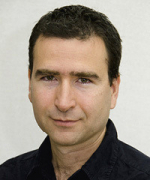Yaron Ostrover, Tel Aviv University
On symplectic inner and outer radii of some convex domains

Symplectic embedding problems are at the heart of the study of symplectic topology. In this talk we discuss how to use integrable systems to compute the symplectic inner and outer radii of certain convex domains.

The talk is based on a joint work with Vinicius Ramos.

### Video

Ostrover slides.pdf

## 03/11/2020, Tuesday, 17:00–18:00 Europe/Lisbon — Online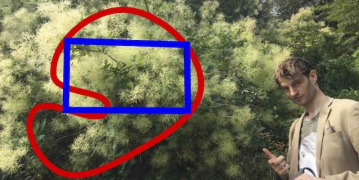Andrew Lobb, Durham University
The rectangular peg problem

For any smooth Jordan curve and rectangle in the plane, we show that there exist four points on the Jordan curve forming the vertices of a rectangle similar to the given one.

Joint work with Josh Greene.

## 10/11/2020, Tuesday, 17:00–18:00 Europe/Lisbon — OnlineEmilio Franco, Instituto Superior Técnico and CAMGSD
Torsion line bundles and branes on the Hitchin system

The locus of the Higgs moduli space fixed under tensorization by a torsion line bundle a key role in the work of Hausel and Thaddeus on topological mirror symmetry. We shall describe the behavior under mirror symmetry of this fixed locus.

Franco.pdf

## 17/11/2020, Tuesday, 17:00–18:00 Europe/Lisbon — OnlineSilvia Anjos, Instituto Superior Técnico and CAMGSD
Loops in the fundamental group of $\mathrm{Symp}(M,\omega)$ which are not represented by circle actions

It was observed by J. Kędra that there are many symplectic $4$-manifolds $(M, \omega)$, where $M$ is neither rational nor ruled, that admit no circle action and $\pi_1 (\operatorname{Ham}( M))$ is nontrivial. In the case $M={\mathbb C\mathbb P}^2\#\,k\overline{\mathbb C\mathbb P}\,\!^2$, with $k \leq 4$, it follows from the work of several authors that the full rational homotopy of $\operatorname{Symp}(M,\omega)$, and in particular their fundamental group, is generated by circle actions on the manifold. In this talk we study loops in the fundamental group of $\operatorname{Symp}_h({\mathbb C\mathbb P}^2\#\,5\overline{\mathbb C\mathbb P}\,\!^2)$ of symplectomorphisms that act trivially on homology, and show that, for some particular symplectic forms, there are loops which cannot be realized by circle actions. Our work depends on Delzant classification of toric symplectic manifolds and Karshon’s classification of Hamiltonian circle actions.

This talk is based in joint work with Miguel Barata, Martin Pinsonnault and Ana Alexandra Reis.

### Video

Silvia slides.pdf

## 24/11/2020, Tuesday, 17:00–18:00 Europe/Lisbon — OnlineLeonardo Macarini, Instituto Superior Técnico and CAMGSD
Dynamical implications of convexity beyond dynamical convexity

We will show sharp dynamical implications of convexity on symmetric spheres that do not follow from dynamical convexity. It allows us to furnish new examples of dynamically convex contact forms that are not equivalent to convex ones via contactomorphisms that preserve the symmetry. Moreover, these examples are $C^1$-stable in the sense that they are actually not equivalent to convex ones via contactomorphisms that are $C^1$-close to those preserving the symmetry. Other applications are the multiplicity of symmetric non-hyperbolic closed Reeb orbits under suitable pinching conditions and the existence of symmetric elliptic periodic Reeb orbits.

This is ongoing joint work with Miguel Abreu.

### Video

Macarini slides.pdf

## 15/12/2020, Tuesday, 17:00–18:00 Europe/Lisbon — OnlineSimon K. Donaldson, Simons Center for Geometry and Physics Stony Brook and Imperial College London
Co-associative fibrations of $G_{2}$-manifolds and deformations of singular sets

The first part of the talk will review background material on the differential geometry of $7$-dimensional manifolds with the exceptional holonomy group $G_{2}$. There are now many thousands of examples of deformation classes of such manifolds and there are good reasons for thinking that many of these have fibrations with general fibre diffeomorphic to a $K3$ surface and some singular fibres: higher dimensional analogues of Lefschetz fibrations in algebraic geometry. In the second part of the talk we will discuss some questions which arise in the analysis of these fibrations and their "adiabatic limits". The key difficulties involve the singular fibres. This brings up a PDE problem, analogous to a free boundary problem, and similar problems have arisen in a number of areas of differential geometry over the past few years, such as in Taubes' work on gauge theory. We will outline some techniques for handling these questions.